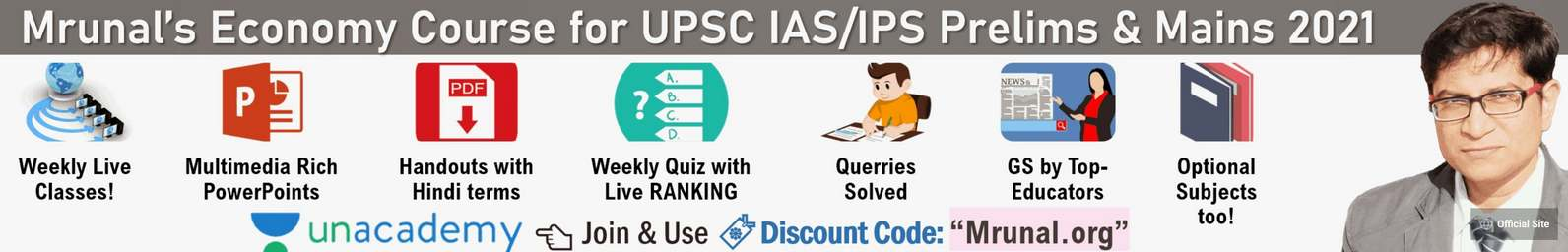## [Studyplan] CSAT Aptitude Paper 2: Maths & Data Interpretation-High priority topics, Sample Questions, free studymaterial (part 2 of 3)

Math haters are grave diggers #1: 2012 unlikely to repeat #2: GS Strength doesn’t compensate math weakness #3: One lakh Delhi aspirants #4: Maths = competitive edge + peace of mind Mathematics: What to ignore? Doubtful syllabus topics in [...]

## [Studyplan] CSAT Aptitude Paper 2: Decision Making + Maths Medium & Low priority topics, Sample Questions, free studymaterial (part 3 of 3)

Decision Making Problem solving & communication Bad decision choices Good decisions Just eliminate two bad decision CSAT 2013 Questions Elimination doesn’t always work though! Where to get practice? Maths: Medium priority topics M1: Arithmetic Progression (AP) M2: Coordinate Geometry [...]

## [Studyplan] CSAT Aptitude Paper 2: Comprehension & Reasoning, analysis, previous papers, free studymaterial, cutoffs (part 1 of 3)

Chart: Analysis of all CSAT papers so far [Section I] Comprehension for CSAT Step1:  Finish theory How to prepare high level reasoning? Step#2: Move hands while reading passage Step#3: Maximum practice Comprehension: Why read Non-GS columns? What about the [...]

## [Reasoning] Calendar Questions: Finding day or date, concepts, shortcuts explained

Introduction Concept: 7 Day cycle SSC-CGL 2000 Question on 7-Day cycle Concept: Day Gain Day loss What is Leap year? SSC Investigator 2010 (Anniversary) SSC CGL 2011 (Anniversary) CAT-2001 Question on calendar Question 1988 to 1989 Speed Technique tip#1 [...]

## [Trigonometry] Finding Minimum Maximum Values for SSC CGL Made Easy without differentiation

This is a guest article by Mr.Dipak Singh. if the math-equations/signs are not clearly visible in your browser, then click me to download the PDF version of this article. Trig-Identities Min-Max table Ratta-fication formulas The AM GM Logic Find [...]

## [Reasoning] Logical Connectives (if, unless, either or) for CSAT, CAT shortcuts formulas approach explained

Difference: Syllogism vs Logical connectives Standard format: logical connectives Logical connective: if then Logical connective: Only IF Logical Connective: UNLESS Logical connective: otherwise Logical connective: When, Whenever, every time Logical Connective: Either OR Demo Q: Only if: bored TV [...]

## [Trigonometry] Type#4: Questions based on Trig and Algebra combo

Algebra: 3 formulas Trigonometry: Formulas Case: Only trig formulas Case: Combo of Trig + algebra formulas Mock Questions Before moving to the next topic of trigonometry, I would like to quote the tweets of SSC chairman’s official account: What [...]

## [Trigonometry] Type#3: Complimentary Angles related Questions and approach for SSC CGL

What is complimentary angles? SIN vs COS SEC vs COSEC TAN vs COT Multiplication chain (tan and cot)  Finding unknown angle The half angles Summary Mock question What is complimentary angles? If you add two angles (A+B) and if [...]

## [Trigonometry] Type#2: Table based questions, memorization technique, approach explained for SSC CGL

Introduction Table based Questions Case1: finding value Case2: multiplication chain Case3: finding angle Mock Questions Introduction For SSC CGL trigonometry, there are mainly four types of questions Height and distance: and it has five subtypes. We already saw how [...]

## [Reasoning] 4-Statement Syllogism: Approach, Techniques, explained for SBI PO (High level reasoning) and UPSC CSAT paper 2

Introduction Recap: 2 statement Complimentary case Approaching 4 statement syllogism Case#1 : Stick, lamps, power, dresses, shirts Case#2: Bird, Horse, Tiger, Lion & Monkey Case#3: Bench, Wall, House, Jungle, Road Case#4: (Complimentary Pairs): Cups, Bottles, Jugs, Plates & Tables [...]

## [Aptitude] LCM, HCF, GCD: Basic concept, calculation, applications explained

Introduction What is Prime number? What is LCM? LCM4 EXam How to find LCM using Prime-Factorization? LCM of two numbers (56, 96) LCM of three numbers: (12,15,20) LCM of prime numbers LCM of co-prime numbers What is HCF or [...]